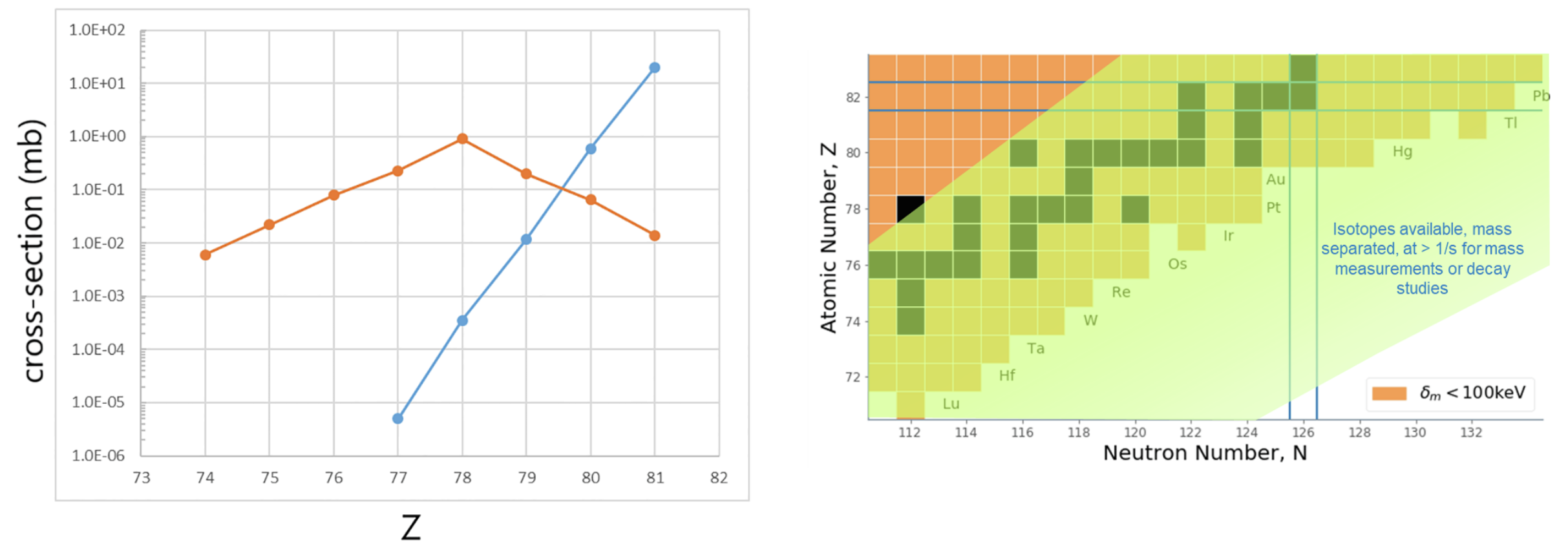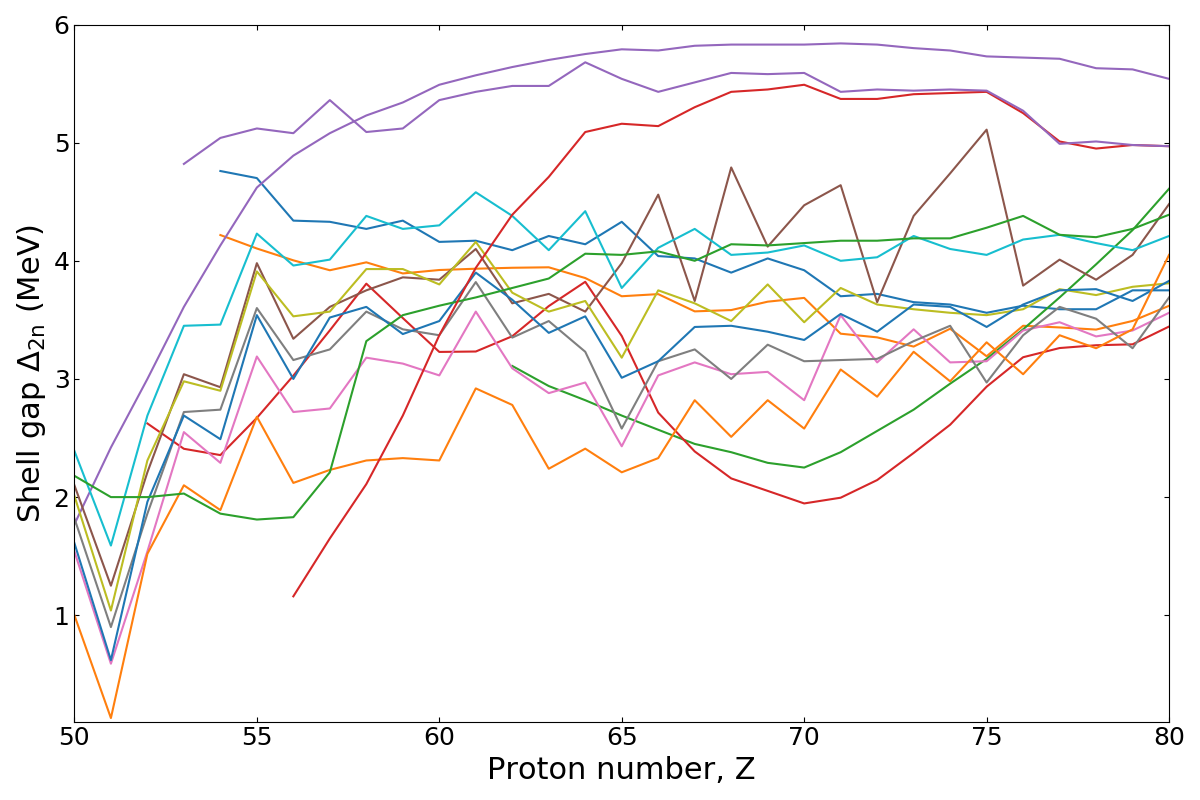## Insights into the $N=126$ Shell ClosureLA-UR-19-25586

### Matthew Mumpower

Workshop on Nuclear Astrophysics Opportunities at ATLAS

Saturday July 13$^{th}$ 2019FIRE Collaboration
Fission In R-process Elements

## Why is the $N=126$ shell closure interesting?

The evolution of nuclear structure throughout the chart of nuclides

GT or FF contributions could dominate in this region for nuclear $\beta$-decay

For the study of reactions ($\gamma$-strength and nuclear level densities)

Formation of the elements in astrophysical environments ($r$-process)

How much actinides are produced in nature? What about superheavies?

Important for high opacity elements (both lanthanides & actinides) that can impact light curvesMorales et al. PRL (2014) • Zhang et al. PRL (2019) • Wu et al. PRC (2019) • Taniuchi et al. Nature (2019) • Figs. from Savard et al. (2019)

## The $N=126$ shell gapThe shell gap can be used as a measure of the strength of a shell closure

A strong shell closure is baked into present nuclear models ($\Delta_{\rm 2n}$ rather flat and $> 0$)

For nucleosynthesis this means it takes awhile to get beyond this hangup spot

Calculation by Mumpower

## Importance for the $r$-process

A dirty secret: nucleosynthesis simulations have trouble reproducing
(1) the peak height (2) peak height relative to $A=130$ and (3) the position of the $A=195$ peakFinal abundances using 20 mass models given the same astro. conditions

The $N=126$ shell closure acts as the gatekeeper to actinide production

If fission recycling: implications for lanthanide production and galactic chemical evolution

Côté et al. ApJ (2018) • Holmbeck et al. ApJ (2019) • Calculation by Mumpower

## The $N=126$ shell closureFig. from teh interwebs

## Summary

The $N=126$ factory will give much needed insight to studies of nuclear structure

A strong $N=126$ shell closure is baked into current nuclear models

This has important implications for the $r$-process of nucleosynthesis...

The $N=126$ closure acts as the gatekeeper to actinide production

Astro. observations are influenced depending on production of high opacity elements

Even lanthanide production is influenced if fission recycling is active

This is important if we want to understand the history of element production in our galaxy

Results / Data / Papers @ MatthewMumpower.com